### Conversion From Three Phase to Tow phase (Scott Connection)

With the help of Scott connection, proposed by C.F. Scott, it is possible to obtain 2phase supply which is required for furnaces or even three phase load can be driven from the available 2phase supply source. The Scott connection which is serving this purpose is shown in the Fig.1.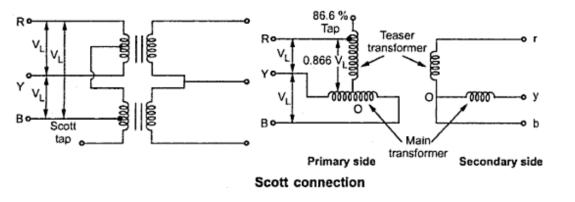Fig.  1
This connection uses tow transformers with different rating. But identical transformers with suitable tapping may also be used for the interchangeability and provision of spares. One of the transformers having 50% taping is called main transformer and other one having 86.6% tapping is called teaser transformer. If the secondaries of the tow transformers are connected as shown in the Fig. 1 then tow phase, three wire system is obtained.
One end of the primary winding of the teaser transformer is connected to the centre tapping provided on the primary winding of the main transformer.The tow ends of the primary winding of the main transformer and 86.6 tapping point on the teaser transformer is connected to a balanced three phase supply. The voltage per turn is same both in primary of both main and teaser transformer. With the equal number of turns on secondaries of both the transformers, the secondary voltage will be equal in magnitude which results in symmetrical 2phase system.
The same connection drawn slight differently is shown in the Fig. 2.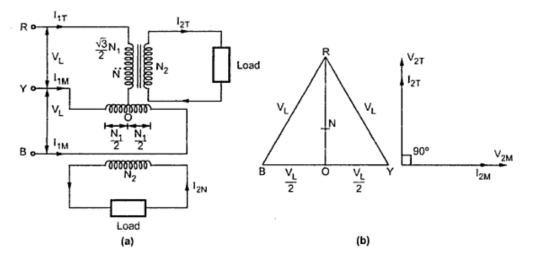Fig.  2
The main transformer primary winding consists of N1 turns connected between lines Y  and B of a symmetrical three phase supply. VRY, VYB and VBR are all line voltages. Hence VRY= VYB = VBR = VL . But RO being the altitude of the equilateral triangle, the voltage VRO is (√3/2)VL. The voltage per turn will be same in primaries of both the transformers if number of turns between R and O are (√3/2)N1. With this then the terminal voltages on the secondary windings having same turns will be equal in magnitude and have a same difference of between them.
The point O is not the neutral point of  3 phase supply voltage as its voltage with respect to any line is not VL/√3. N is the neutral point shown in Fig. 3. Voltage VRN is nothing but VL/√3 whereas VRO is (√3/2)VL. Hence the voltage between N and O will be

(√3/2)VL - (1/√3)VL = 0.288 V ~  0.29VL

Since 0.29 is one third of 0.866, N divides the teaser winding RO in the ratio 2 :1.
Now let us consider the unity power factor load. The teaser secondary is supplying a current of I2T . Neglecting the magnetizing current we have IO we have,
Transformation ratio,                        K = I1T/I2T = N2 /N1

Consider,                                         I1T/I2T = N2/N1
I1T = I2T x (N2 /((√3/2)N1)) = (2/√3) (N2/N1) I2T
=1.15 ( N2/N1) I2T
...                                                      I1T = 1.15 K I2T                                           ..................(i)
Each half on the primary winding of the main transformer carries current of consisting of I1m tow parts.
i) First part balances the main secondary current
I1M = ( N2/N1) I2M = K I2M

ii) The second part is equal to one half of the teaser primary current i.e. 0.5 I1T
The main transformer primary winding forms a return path for the teaser primary current which is divided into tow halves at point O in either direction.
The current in each half is equal to (I1T/2)=((1.15 K I2T ) /2) = 0.58 K  I2T
This current is shown in the Fig. 3Fig.  3
Thus the phase R supplies current I1T which is divided into equal parts and is flowing in the main transformer in the opposite directions. Thus the line currents on the primary side are vectorially given by,

ĪR = Ī1T
ĪY = Ī1M - 0.5 Ī1T = Ī1M - 0.5 Ī1T
ĪB = -Ī1M - 0.5 Ī1T = - Ī1M - 0.5 Ī1T
The magnitude of these line currents are given by,

IR = I1T
IY = IB = √(( I1M )2 + (0.5 I1T )2)

The currents in the lines Y and B are obtained vectorially. The teaser transformer currents flowing in the tow halves of the primary winding of the main transformer in the opposite direction and have no magnetic effect on the core and does not take part in balancing the secondary ampere turns of the main transformer. Thus when tow phase load of unity power factor is balanced then three phase side is also balanced.
Now let us consider balanced row phase load at a lagging p.f. of cosΦ. The corresponding phasor diagram is shown in the Fig. 4. The three phase side is again balanced as the currents drawn from the three phase system are equal balanced and lag by angle Φ with respect to their respective phase voltages. This can be shown mathematically.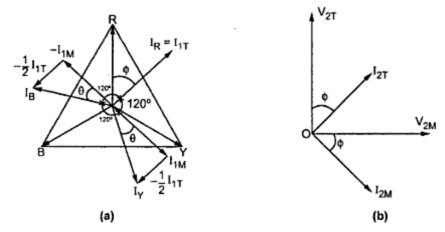Fig.  4
Let us consider the equal currents at a power factor of cos Φ lagging from secondary side
...                    I2T = I2M =  I2
From the phasor diagram we have
IR = I1T
IY = IB = √(( I1M)2 + ( 0.5 I1T)2)
Now,          I1M = K I2M = K I2
I1T = 1.16 K I2T = 1.16 K I2
Substituting         IR = 1.16 K I2
IY = IB = √((K I2)2+(0.5 x1.16 I2)2)
= 1.16 K I2
Thus all the currents in the primary side are equal in magnitude.
We have from the phasor diagram

Since the power factors of the load for the main transformer and  the teaser transformer are equal, the phase angle between the secondary currents is also 90°. The angle between the primary currents I1T and I1M is also 90°. Thus the three line currents IR ,IY and IB are displaced from each other by 120° and lag the respective phase voltages by an angle Φ. This proves that if the tow phase load is balanced then the loading on the three phase side is also balanced.
Now we will consider the case of unbalanced tow phase load having different currents and different power factors. The phasor diagram for this case can be constructed in a similar manner. It is shown in the Fig. 5.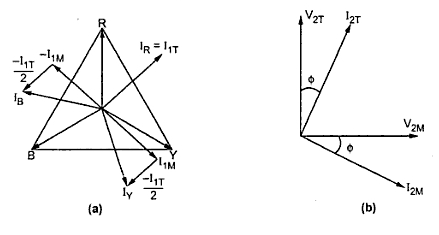Fig.  5
Example  1 :  Two transformers are required for a Scott connection operating from a 415 V, 3 phase supply for supplying two single phase furnaces 225 V on the two phase side. If the total output is 150 KVA, calculate the secondary to primary turns ratio and the winding currents for each transforme.

Solution : For the main transformer
Primary voltage = 415 V
Secondary voltage = 225 V
Transformation ratio,           K = Secondary voltage / Primary voltage = 225/415 = 0.5421
Turns ratio = N2/N1 = 0.5421
Secondary current = (150 x 103)/( 2 x 225) = 30 A
Primary current = 300 x 0.5421
= 162.63 A
For teaser transformer
Primary voltage = (√3/2) x 415 = 359.40 V
Secondary voltage = 225 V
Transformation ratio,               K = Secondary voltage/ Primary voltage = 225/359.4 = 0.6260
Turns ratio,                              K = N2/N1 = 0.6260

Example 2 : In a Scott connection, calculate the values of line currents on the three phase side, if the loads on 2 phase side are 300 kW and 400 kW, both at 125 V and 0.707 p.f. and the three phase line voltage is 3300 V. The 300 kW load is on the leading phase of the 2 phase side. Neglect the losses.

Solution :

Primary line voltage = 3300 V
Secondary voltage = 125 V
Load carried by teaser secondary = 300 kW at 0.707 p.f.
Load carried by main secondary = 400 kW at 0.707 p.f.
Transformation ratio for main transformer, K =V2 /V1 = 125/3300 = 0.0378
Transformation ratio for teaser transformer, K = 1.1547 x Transformation ratio for main transformer
= 1.1547 x 0.0378 = 0.0436
Power supplied by teaser transformer = 300 kW at 0.707 p.f.
Secondary current in teaser transformer, I2T = (300 x 103) / (125 x 0.707) = 3394.62 A
Primary current in teaser transformer, = Transformation ratio for teaser transformer  x I2T
= 0.0436 x 3394.62 = 148 A
...     Line current,                                             IR = 148 A
Power supplied by main transformer = 400 kW at 0.707 p.f.
Secondary current in main transformer, I2M= (400 x 103) / (125 x 0.707) = 4526.16 A
Primary current in main transformer, I1M= K . I2M
= 0.0378 x 452.16 = 171.08 A
In addition to this current each half of the primary winding of the main transformer carries half of teaser primary current.
I1T = 148 A ;  I1T /2 = 148/2 = 74 A
Total current carried = √((I1M)2 + (I1T /2)2) = √((171.08)2 + (74)2) = 186.39 A
IY = IB = 186.39 A
The three line currents are IR = 148 A, IY = 186.39  A, IB= 186.39 A

Related articles :

1.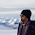2.